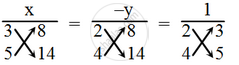# Solve the following system of equations by cross-multiplication method. 2x + 3y + 8 = 0; 4x + 5y + 14 = 0 - Mathematics

Sum

Solve the following system of equations by cross-multiplication method.

2x + 3y + 8 = 0

4x + 5y + 14 = 0

#### Solution

The given system of equations is

2x + 3y + 8 = 0

4x + 5y + 14 = 0

By cross-multiplication, we get\Rightarrow \frac{x}{3\times 14-5\times 8}=\frac{x}{3\times 14-5\times8}=\frac{1}{2\times 5-4\times 3}

\Rightarrow \frac{x}{42-40}=\frac{-y}{28-32}=\frac{1}{10-12}

\Rightarrow \frac{x}{2}=\frac{-y}{-4}=\frac{1}{-2}

\Rightarrow \frac { x }{ 2 } = \frac { -1 }{ 2 }

⇒ x = – 1

\Rightarrow \frac { -y }{ -4 } = \frac { -1 }{ 2 }

⇒ y = – 2

Hence, the solution is x = – 1, y = – 2

We can verify the solution.

Concept: Algebraic Methods of Solving a Pair of Linear Equations - Cross - Multiplication Method
Is there an error in this question or solution?